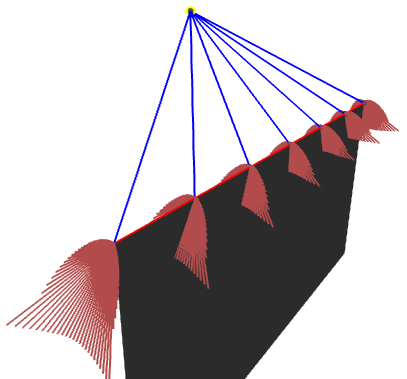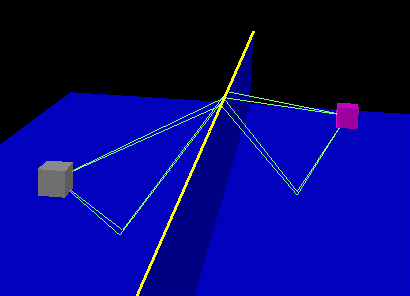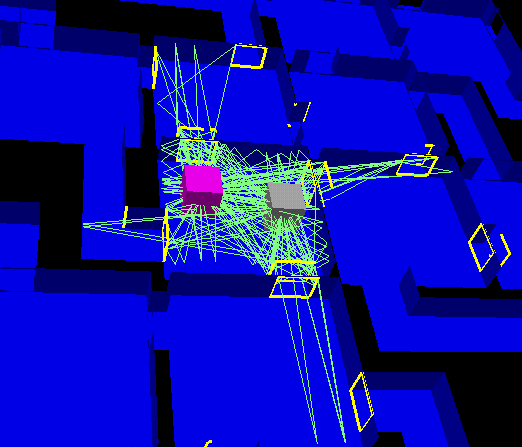Real-Time Geometrical Theory of Diffraction

Geometrical theory of Diffraction is an extension of geometrical optics aimed at taking into account diffraction i.e.
light/sound propagating in the geometrical shadow regions of an obstacle. We designed a system that can construct
and evaluate in real-time the contribution of any hybrid reflection/diffraction path in a general 3D environment.

Results

Visualizing the diffraction coefficient
The following picture shows the amplitude of the diffraction coefficient computed using uniform GTD in the case
of a half-screen diffraction.Building the diffraction paths
The following picture shows all hybrid specular reflection/diffraction paths between a point source and
a point receiver. The highlighted edge is the source of the diffraction.A more complex example, showing all diffracting edges visible from the receiver (in magenta)
either directly or through one specular reflection and all hybrid diffracted/reflected path for 1 order of reflection
and 1 diffraction only !!!Related publications